# Catch up on the latest AI articles# Can Reinforcement Learning Solve Complex Tasks On Real Robots?

3 main points
✔️ Propose a method to solve complex manipulation tasks on real machines by combining Feedback Controller and RL
✔️ We show that the method is robust to uncertainties such as noise.
✔️ Achieves high sample efficiency and performance compared to RL alone

written by Tobias JohanninkShikhar BahlAshvin NairJianlan LuoAvinash KumarMatthias LoskyllJuan Aparicio OjeaEugen SolowjowSergey Levine
(Submitted on 7 Dec 2018 (v1), last revised 18 Dec 2018 (this version, v2))
Subjects: Robotics (cs.RO); Machine Learning (cs.LG)code：The images used in this article are from the paper, the introductory slides, or were created based on them.

## first of all

Robots are used to perform repetitive tasks in manufacturing, but they are vulnerable to a variety of tasks and uncertainties. One of the most commonly used methods to control robots is PID control, which allows the robot to follow a predetermined trajectory. Many manufacturing tasks require adaptation to uncertainty and feedback to the environment, and designing a feedback controller to handle this is very expensive. This is because the feedback controller must be designed not only to follow the trajectory, but also to take into account the contact with the object and the friction caused by the contact, etc. Even if a suitable physical model is given, it is very difficult to determine the physical parameters such as the contact. Another drawback of using such a controller is that it is not very versatile, since it works within a pre-designed range.

Reinforcement learning (RL), on the other hand, learns by interacting with the environment, so it can solve tasks that involve contact, and it also has some promise for versatility. However, RL has a problem in that it requires a large number of samples to train a robot, and it is very difficult to train a robot using actual machines. In addition, RL requires a lot of exploration for learning, but there is a safety problem in experiments on actual machines. Therefore In this paper, we deal with control problems that are difficult to solve with conventional feedback controllers. The main contribution of this paper is to show how to solve the problem using RL. The main contribution of this paper is to propose a method to combine the conventional feedback controller and RL. This article introduces this method and its performance in experiments on actual machines.

## technique

So how do you combine a traditional feedback controller with RL? The figure below shows a rough diagram of the method. As shown in the figure below, the robot learns to maximize the reward by adding the actions of the policy learned by RL and the actions of the controller defined in advance, and the result is used as the robot's action. The figure on the right shows an assembly task solved using this method. This is the base of the method, and we will explain it in more detail in this chapter.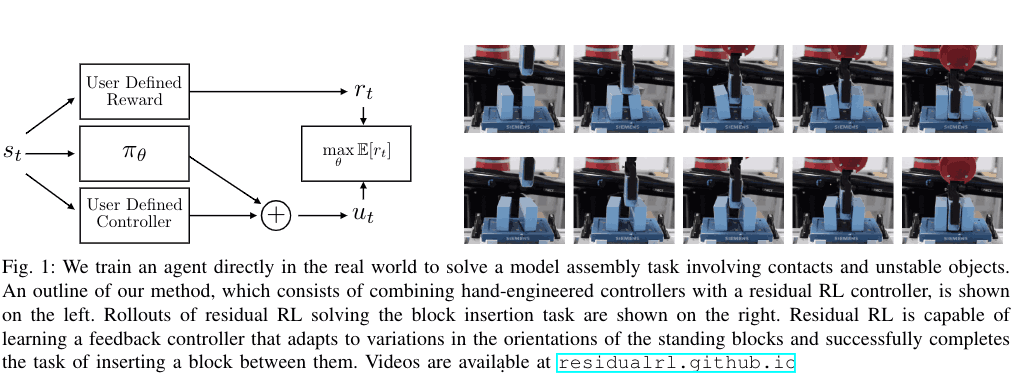### Residual Reinforcement Learning

For most robot-based tasks, the reward function can be thought of as follows.

$$r_{t} = f(s_{m}) + g(s_{o})$$.

Here, $s_{m}$ represents the robot state and $s_{o}$ represents the object state. The $f(s_{m})$ represents the geometric relationship between the robot states, e.g., the reward for moving the robot's gripper closer to the object to be manipulated. And $g_{s_{o}}$ is the reward for the state of the object, for example, the reward for keeping the object vertical.

When we divide them in this way, the conventional feedback controller is suitable for optimizing $f_{m}$, while RL is suitable for optimizing $g(s_{o})$ which involves contact with an object. In order to take advantage of these two advantages, we make the ACTION of AGENT as follows.

$$u=\pi_{H}(s_{m}) + \pi_{\theta}(s_{m}, s_{o})$$.

where $\pi_{H}(s_{m})$ represents the controller designed by humans, $\pi_{\theta}(s_{m}, s_{o})$ is the policy learned by RL and $\theta$ represents the parameters. The designed feedback controller $\pi_{H}$ is able to optimize $f(s_{m})$ quickly, and thus achieve higher sample efficiency. In addition, the errors generated by the feedback controller can be compensated by the Residual RL, which finally allows the task to be solved.

The following shows the algorithm of the method and we used Twin delayed deep deterministic policy gradients (TD3) as RL method for training.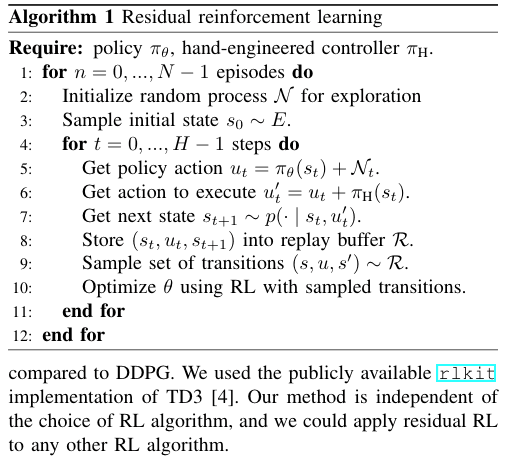## experiment

In this experiment, we will confirm the following.

- Whether the use of hand-designed controllers improves RL sample efficiency and performance?　Also, whether recovery can be performed for incomplete hand-designed controllers?
- Whether the proposed method can be solved for a variety of environments? (generality)
- Whether the proposed method can solve the task in a noisy system?

To verify these results, we conducted experiments on the Assembly task in both a simulation environment and an actual machine environment. The goal of both is to solve the same task, which is to insert the block that the robot's gripper is holding between two blocks. In the following sections, we will introduce the different settings for the simulation and actual environments.

Simulation Environment: In the simulation environment, the Cartesian-space position controller was used to control the robot. In order to allow the insertion of blocks, we made sure that the two blocks have enough space between them. The reward function is represented as follows.

$$r_{t} = -||x_{g} - x_{t}||_{2} - \lambda (||theta_{l}||_{1} + ||\theta_{r}||_{1})$$.

Where $x_{t}$ represents the current block position, $x_{g}$ represents the goal position, $\theta_{l}$ and $\theta_{r}$ represent the left and right block angles (y-axis), and $\lambda$ represents the hyperparameter.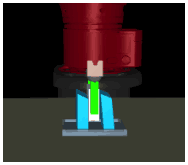Real environment: In the real experiments, we used a compliant joint-space impedance controller as the robot controller. The task is the same as in the simulation environment, but instead of receiving the ground truth position coordinates of objects, etc., the agent receives the estimated coordinates obtained by using a camera tracking system. We used the following reward functions.

$$r_{t}=-\|x_{g}-x_{t}|_{2}-\lambda(\|\theta_{l}|_{1}+\|\theta_{r}|_{1})$$

$$-\mu\|X_{g}-X_{t}\|_{2}-\beta(\|\phi_{l}\|_{1}+\|\phi_{r}\|_{1})$$

where $x_{t}$ are the coordinates of the end effector, $x_{g}$ are the coordinates of the goal, $X_{t}$ are the current coordinates of both standing blocks, $X_{g}$ is the desired position of the object, $\theta_{l}$ and $\theta_{r}$ are the angles of the right and left objects with respect to the y-axis direction, and $\ phi_{l}$ and $\phi_{r}$ are the angles of the right and left objects with respect to the z-axis direction. The $\lambda$, $\mu$, and $\beta$ represent hyperparameters, respectively.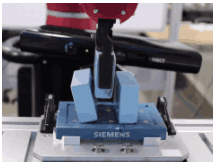In this experiment, we compared the case where the task was solved using only RL (Only RL) and the proposed method, Residual RL, as a comparison.

### Residual RL sample efficiency

The following figure shows the experimental results of Only RL and Residual RL in the simulation environment and the actual machine environment. As you can see in the figure below, the Residual RL performs better in both cases, and has a higher success rate with fewer samples, suggesting that it is more sample efficient. This is because, unlike Residual RL, Only RL has to learn the problem of position control from scratch through interaction with the environment, which results in poor sample efficiency. In particular, the issue of sample efficiency is very important in experiments using actual machines, and therefore Residual RL is particularly suitable for solving problems using actual machines.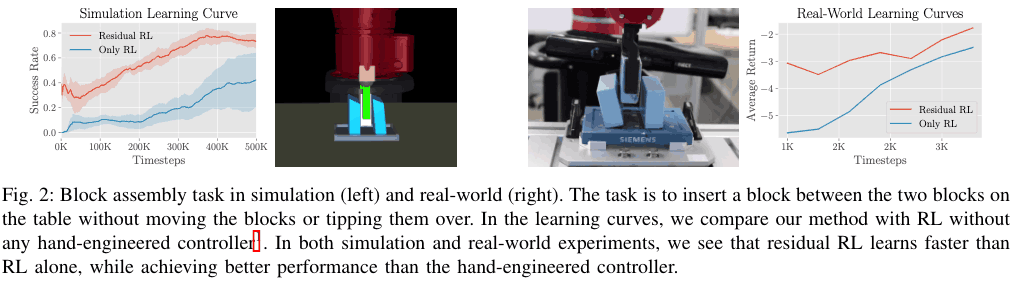### Effectiveness in a variety of environments

If there is enough space between the two blocks and there is no error in the initial positions of the two blocks, the hand-engineered controller can solve the problem with a high success rate. On the other hand, when there is an error, it is difficult to solve with the hand-engineered controller, and the success rate is 2/20, as shown in the table below (a). This is due to the fact that when solving, in order to insert the block that the gripper is holding in its hand, the the block that is being placed. This would be very difficult to do manually with a hand-engineered controller, but Residual RL was able to handle the situation. The result is shown in the graph (b) below. The fact that we were able to train 8000 samples on the actual machine in only 3 hours shows the high sample efficiency.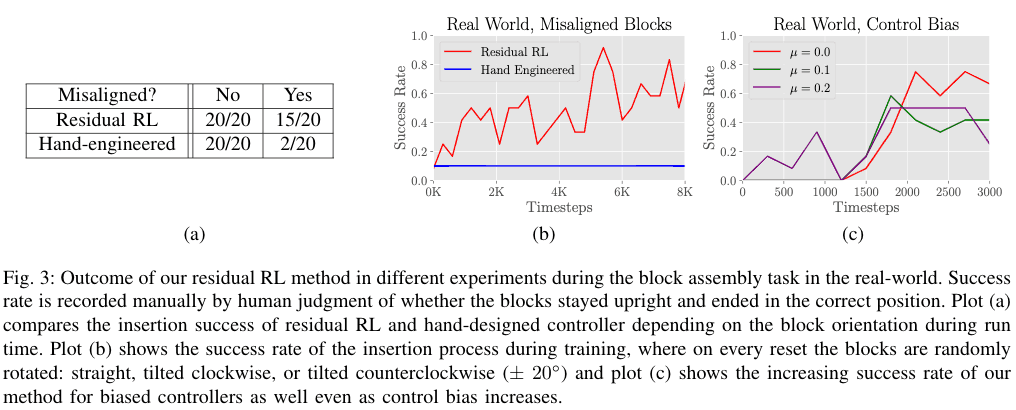### Recovering from control noise

Here we compare the Residual RL with the hand-engineered controller alone when using a controller with a bias and when control noise is present. In (b) below, when control noise is included, the reward of the hand engineered controller drops significantly, while the reward of the Residual RL does not decrease much, indicating that it is more robust to noise. Similarly, when using a controller with a bias, the performance of the hand-engineered controller drops rapidly as the noise increases, while the performance of the Residual RL does not drop. drift and other problems that may occur in actual devices.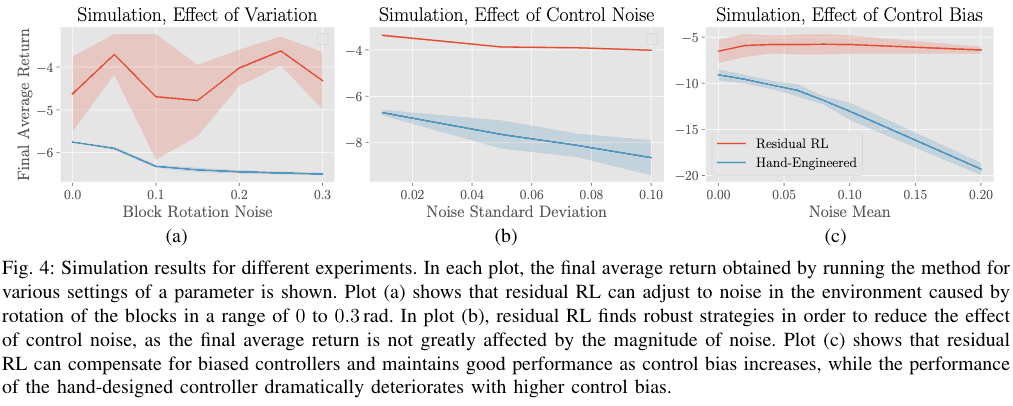### Sim-to-Real in Residual RL

In the Sim-to-Real experiment, we found that when a Residual RL policy is initialized by simulation and then transferred to a real-world environment, it is able to solve the task more quickly than when the Residual RL is trained only on the actual machine or only on the RL. Therefore, it is possible to solve tasks with difficult contacts. Therefore, it is considered to be a very effective method for solving tasks with difficult contacts.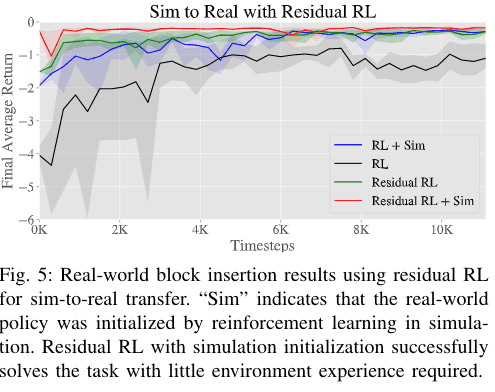## summary

Most of the papers that aim to solve robot tasks using reinforcement learning have because it is difficult to learn on an actual robot due to sample efficiency issues. In this paper, we propose a new method to improve the sample efficiency. In this paper, we combine the conventional feedback controller with reinforcement learning in order to improve the sample efficiency and to enable learning on the actual machine. Recently, there are more and more papers that aim to improve the sample efficiency by combining RL with conventional methods, such as combining motion planning and RL. In the future, I feel that it will be important to explore how RL can be used most effectively in actual machines rather than in simulations.

If you have any suggestions for improvement of the content of the article,